## Negative Binomial Distribution

Also known as the Pascal Distribution and Pólya Distribution. The probability of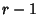successes andfailures in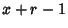trials, and success on theth trial is(1)

where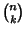is a Binomial Coefficient. Let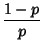(2)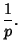(3)

The Characteristic Function is given by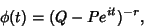(4)

and the Moment-Generating Function by(5)

but, since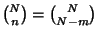,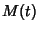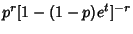(6)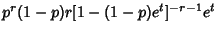(7)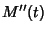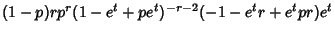(8)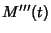(9)

The Moments about zeroare therefore(10)(11)(12)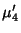(13)

(Beyer 1987, p. 487, apparently gives the Mean incorrectly.) The Moments about the mean are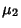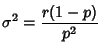(14)(15)(16)

The Mean, Variance, Skewness and Kurtosis are then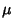(17)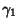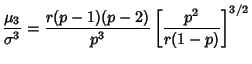(18)(19)

which can also be written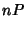(20)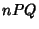(21)(22)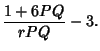(23)

The first Cumulant is(24)

and subsequent Cumulants are given by the recurrence relation(25)

References

Beyer, W. H. CRC Standard Mathematical Tables, 28th ed. Boca Raton, FL: CRC Press, p. 533, 1987.

Spiegel, M. R. Theory and Problems of Probability and Statistics. New York: McGraw-Hill, p. 118, 1992.